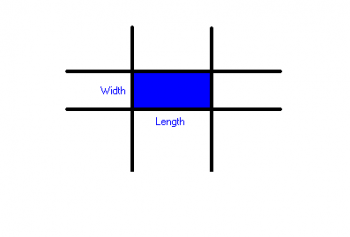Games
Problems
Go Pro!

Problems > Mr. T's Calculus > 2002

## How fast does it change?

A particular dance is performed with four moving poles. At any one instant in time, these poles form a rectangle. The poles forming each pair of opposite sides move closer together and farther apart throughout the course of the dance. The dancer is to step into this rectangle and out again in such a way that he or she never gets pinched by the poles. In the diagram shown, assume that the Length of the rectangle varies according to the equation L = 4 + 3cos t, and the Width of the rectangle varies according to the equation W = 3 + 3sin t, where t is in seconds, and the dimensions are in feet. The area, A, then varies as a function of time.Find the rate at which the area is changing at time t = 5 seconds. Be sure to indicate whether the area is increasing or decreasing.

View the solutionWhat's my equation?What's my distance?Like us on Facebook to get updates about new resources